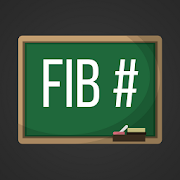## Fibonacci Numbers helps you practice Grade HS Math - Lumos Educational App Store41
Price -fREE
\$0

#### DESCRIPTION:

This app tests to see if a number is a Fibonacci number, and also generates a sequence of Fibonacci numbers.

#### OVERVIEW:

Fibonacci Numbers is a free educational mobile app By .It helps students in grades HS practice the following standards HSF.IF.A.3.

This page not only allows students and teachers download Fibonacci Numbers but also find engaging Sample Questions, Videos, Pins, Worksheets, Books related to the following topics.

1. HSF.IF.A.3 : Recognize that sequences are functions, sometimes defined recursively, whose domain is a subset of the integers. For example, the Fibonacci sequence is defined recursively by f(0) = f(1) = 1, f(n+1) = f(n) + f(n-1) for n ≥ 1..

HS

#### STANDARDS:

HSF.IF.A.3

Developer:

Software Version: 1

Category:

### RELATED APPSEdSearch WebSearch